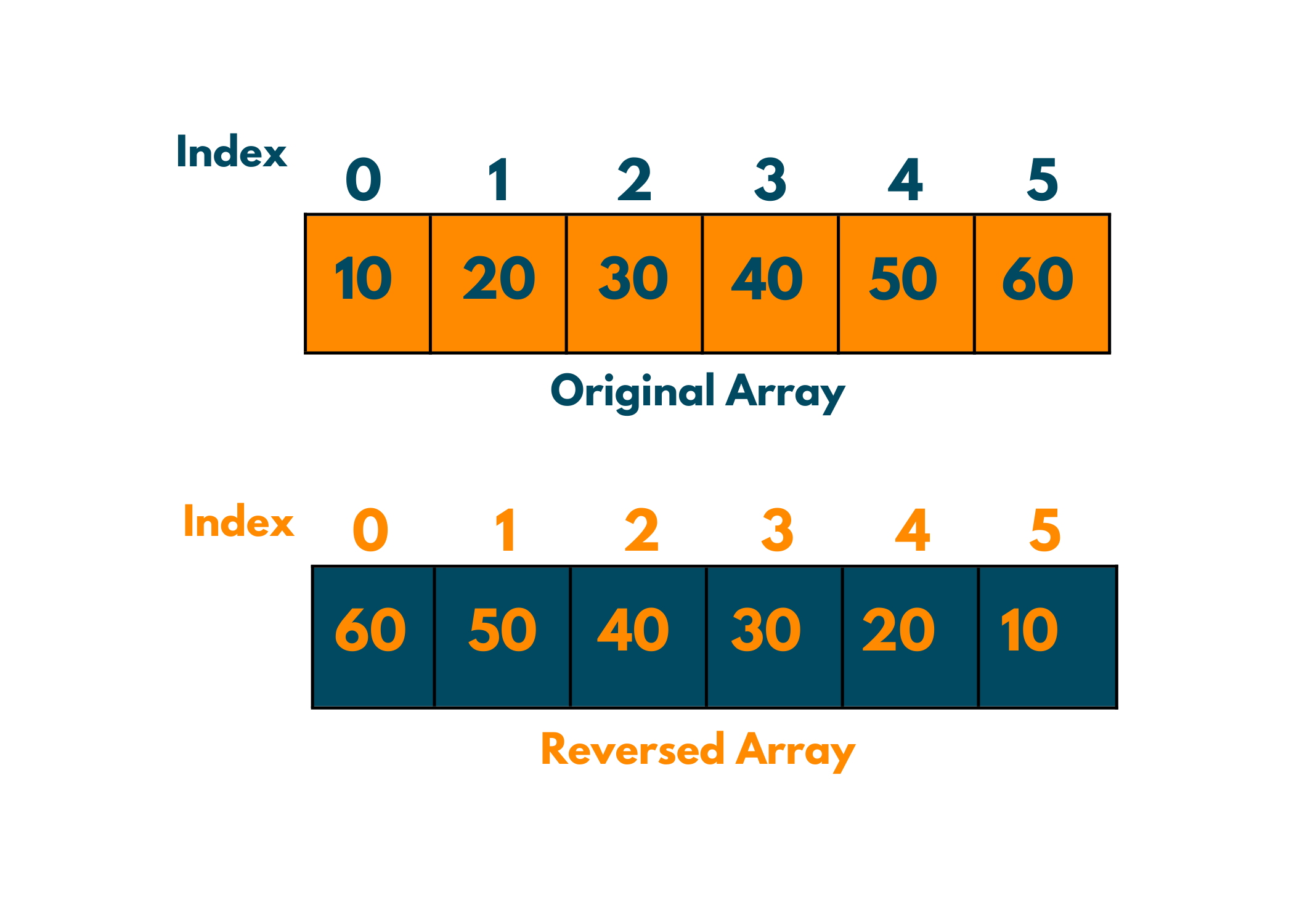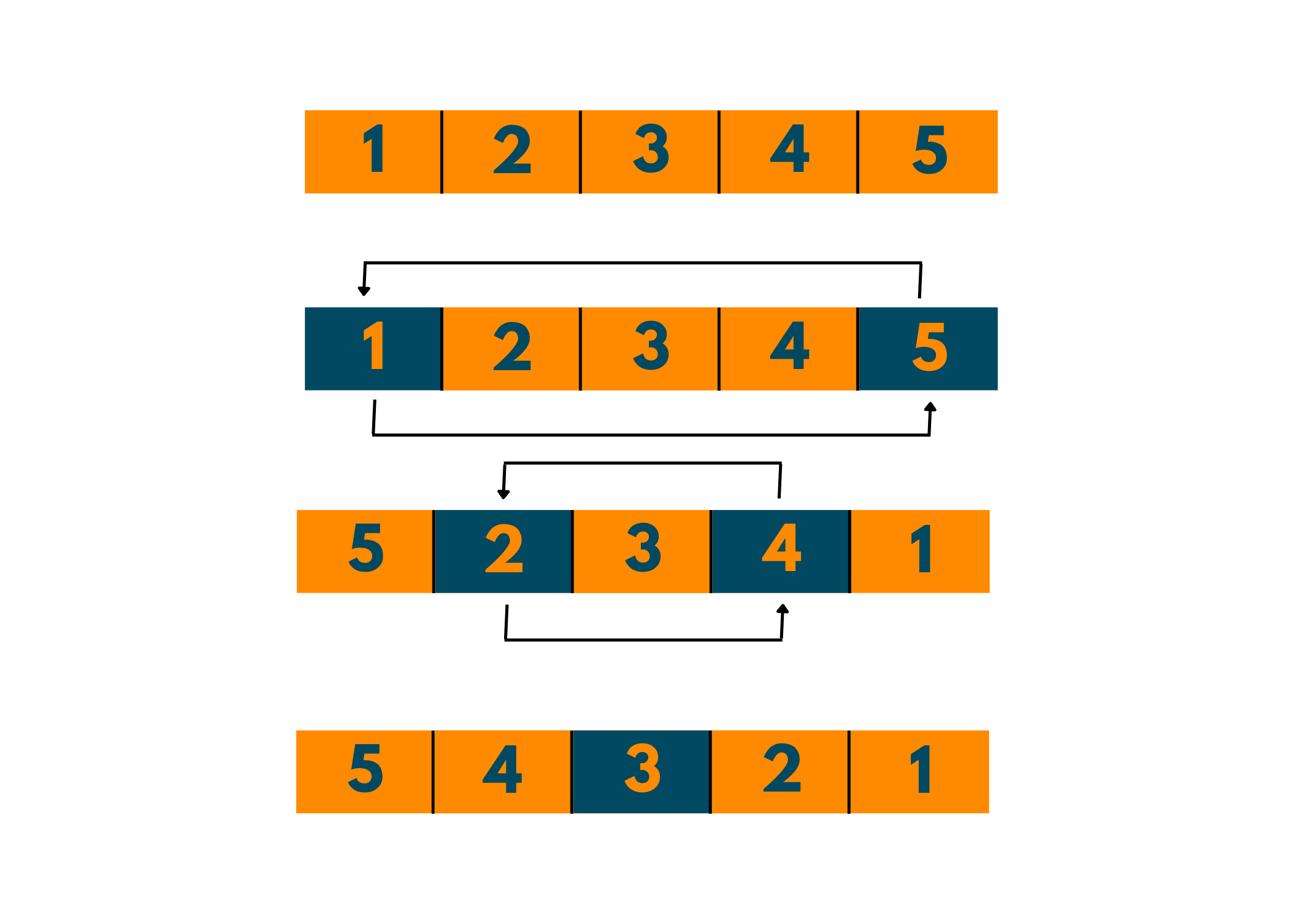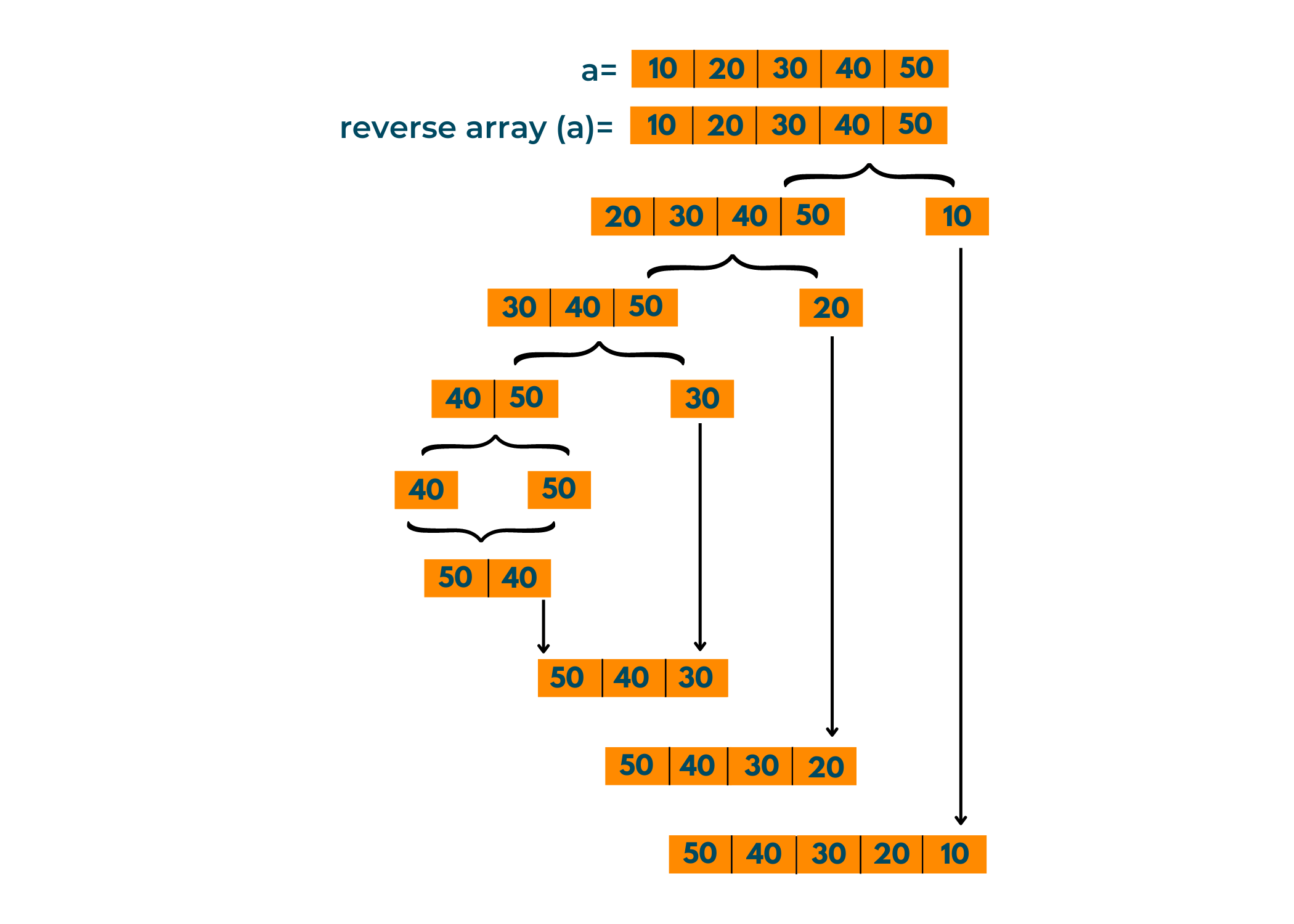# 5 Methods to Reverse Array in Python (reverse, recursion etc)

If you are familiar with other programming languages like C, C++, and Java, you must be aware of the in-built Array data structure. These languages provide the facility to declare and create an Array by defining the required memory size. Python doesn't have an in-built Array data structure, instead, it offers Lists. Arrays and Lists are very different. While arrays have a defined number of elements, Lists are adaptable.

### What does Reversing an Array mean?

There are many problems where reversing an array can be considered a better brute-force solution than any other approach. Sometimes, you might find the need to go through an array from the back. There are many scenarios that might demand the use of a reversed array in Competitive Programming. For example, consider the example of a Palindrome number or printing reverse patterns!

Reversing an array literally means just reversing the order of array elements.

For example - [1,2,3,4]        =>                [4,3,2,1]

Check out the image below to see what reversing an array looks like -Reversing an array is one of the basic functions while programming and Python make it a lot easier!!

### How to Declare an Array in Python?

Before moving on to reversing an array, let's revise how we declare an array in Python. Since Python does not provide in-built arrays, the array module has to be imported in order to use arrays.

```#import the array module
import array as arr

#initializing array with integers
a = arr.array('i', [1,2,3,4,5])
print('The array is: ',a)
```

In order to declare an array, we need to also declare the data type it is storing. (as here integers are expressed with 'i')

Output:

```The array is:  array('i', [1, 2, 3, 4, 5])
```

Now, let's move on to how to reverse an array in Python.

## How to Reverse an Array in Python?

There are many user-defined and in-built methods in Python to reverse an array. Determining the correct optimal algorithm, that is compatible with your code, is really important to avoid errors and save time. Let's look into a few methods in detail.

Note that we are dealing here with Arrays and not Lists!

### Method 01) Swapping the elements

Let's start by reversing an array in Python without using a built-in function. The most common thing you can do is to swap the elements at the opposite/counterpart indexes. For a better understanding, look at the code below:

```# import the array module
import array as arr

# initializing array with integers
a = arr.array("i", [1, 2, 3, 4, 5])
print("The array: ", a)
# get size of the array using len() method
l = len(a)
# going through the array only halfway!!
for i in range(l // 2):
# swap the elements
a[i], a[l - i - 1] = a[l - i - 1], a[i]

print("The reversed array: ", a)
```

The swapping makes it pretty confusing, doesn't it?? Don't worry, take a look at the presentation below:I hope it's easy to understand now.

Have a look at the output for the code above:

```The array:  array('i', [1, 2, 3, 4, 5])
The reversed array:  array('i', [5, 4, 3, 2, 1])
```

This is just an example of how to reverse an array without using any in-built method. Coming forth, you'll see more such methods.

### Method 02) Using reverse() Method

To reverse an array in Python you can use the reverse() method directly on both, lists and arrays. Using the reverse() method is beneficial when you have a memory space constraint since it performs the operation on the same memory and doesn't require any extra space. Remember how we swapped the elements above, without any extra memory. reverse() does almost the same.

The code:

```# import the array module
import array as arr

# initializing array with integers
a = arr.array("i", [1, 2, 3, 4, 5])
print("The array: ", a)

#calling reverse() method on array a
a.reverse()

print("The reversed array: ", a)
```

This is one of the fastest methods to reverse an array in Python. This method is also suitable for long-length arrays.

Output:

```The array:  array('i', [1, 2, 3, 4, 5])
The reversed array:  array('i', [5, 4, 3, 2, 1])
```

The reverse() method is called upon the array (as an object calling a method).

### Method 03) Using reversed() Method

To reverse an array, Python also offers reversed() method. The reversed() method doesn't create any copy of the array, nor does it make any changes in the original array. Instead, it returns an iterator. This method is made to facilitate reverse iteration over sequences.

Check out the code below:

```# import the array module
import array as arr

# initializing array with integers
a = arr.array("i", [1, 2, 3, 4, 5])
print("The array: ", a)

#passing the array in reversed()
reversed(a)

print("The reversed array: ", a)
```

Output:

```The array:  array('i', [1, 2, 3, 4, 5])
The reversed array:  array('i', [1, 2, 3, 4, 5])
```

Notice how there is no change in the original array. Now, let's try storing the reversed(arrayName) into another variable:

```# import the array module
import array as arr

# initializing array with integers
a = arr.array("i", [1, 2, 3, 4, 5])
print("The array: ", a)

#passing the array in reversed()
reversed_a = reversed(a)

print("The reversed array: ", reversed_a)
```

You'll be quite surprised to see the output:

```The array:  array('i', [1, 2, 3, 4, 5])
The reversed array:  <reversed object at 0x0000025EC428EC88>
```

See! The reversed() method returned an iterator, which got stored and printed further. In order to get a reversed list, i.e. a copy of the reverse of the original array, you need to apply list() over the reversed_a variable. Indirectly, you have to convert the iterator to a list in order to get the reversed array.

```# import the array module
import array as arr

# initializing array with integers
a = arr.array("i", [1, 2, 3, 4, 5])
print("The array: ", a)

#passing the array in reversed()
reversed_a = list(reversed(a))

print("The reversed array: ", reversed_a)
```

Now, the reversed array gets stored in reversed_a and hence we obtain a reversed array.

```The array:  array('i', [1, 2, 3, 4, 5])
The reversed array:  [5, 4, 3, 2, 1]
```

You must be wondering what is the difference between the reverse() and reversed() method. Is it just about a 'd'?

No!! Although the reverse() and reversed() perform the same function, they are different. While reverse() works on the same array, without consuming any extra memory space, reversed() needs a new array to store a copy of the reversed array. Also, while the reverse() just reverses the original array, reversed() does not affect the original array and returns an iterator, to reverse iterate over a sequence.

Note that since reversed() returns an iterator, it only works with sequential objects. The reversed() method will not be applicable to sets and iterators.

### Method 04) Using Slicing

This is the most basic and used method for reversing an array. It has no effect on the original array; instead, it returns a reversed copy of the original array. The Slicing method iterates over the array from beginning to end (last index).

How to Slice an Array? Slicing is the selection of a specific part of an array, rather than the entire array. Slicing is the process of selecting/calling a part of an array, given the beginning and ending indexes. To slice an array, the following syntax is followed-

arrayName[start: end: step]

Here, the start represents starting index of your sliced array.

end represents the ending index of the sliced array, (the end is excluded)

the step is an optional parameter, that is generally used when you need to skip a few elements in your slice. For instance, every second element of the slice.

To reverse an array, the step parameter plays the most important role.

For example, slice the array a = [1, 2, 3, 4]

1. from start= 0 to end = 2        =>     a[:3]

2. from start = 2 to rest of the array           =>       a[2:]

3. from start = 1 to rest of the array, skipping the next element        => a[1::1]

You need to pay attention to the index of the array in order to understand Slicing.

In Python, we can also represent the index of the array with negative numbers, where the end of the array is marked by -1.

The syntax of slicing to reverse an array in Python is:

arrayName[::-1]

Since the start index of an array, by default, is 0. Hence adding (-1) to 0, end up with -1, i.e. the last index of the array.

Adding (-1) to (-1) results in (-2), which is the second last index of the array. This continues till it reaches the last (first) index of the array.

Example:

```# import the array module
import array

#function to reverse the array
def reversingArray(a):
return a[::-1]

# declare an array
a = array.array("i", [1, 2, 3, 4, 5])
print('The original array is: ', a)
print('The reversed array is ',reversingArray(a))

reversingArray(a)
#The above statment won't work. Can you guess, why?
print(a)
```

Note that the command reversingArray(a) won't work, since we are returning the array in our function, but we haven't assigned any variable to store the output.

Output:

```The original array is:  array('i', [1, 2, 3, 4, 5])
The reversed array is  array('i', [5, 4, 3, 2, 1])
array('i', [1, 2, 3, 4, 5])
```

Instead of creating a function, you can always use a[::-1] directly.

Another example:

```# import the array module
import array

# declare an array
a = array.array("i", [1, 2, 3, 4, 5])
print('The original array is: ', a)
#slicing the array
print('The reversed array is ', a[::-1])
#printing the original array again
print('Effect on original array: ', a)
```

See! Slicing won't actually reverse the array for you. Instead, it will provide a way to iterate over the array in reverse order.

Output:

```The original array is:  array('i', [1, 2, 3, 4, 5])
The reversed array is  array('i', [5, 4, 3, 2, 1])
Effect on original array:  array('i', [1, 2, 3, 4, 5])```

So, what is the difference between Reversing and Reverse Iteration of an Array? Reversing an Array in Python is to reverse the contents of an array, be it changing the original array or creating a reversed copy of it. Whereas performing reverse iteration over an array simply implies iterating over the loop from the end of the array.

Check out the code below for a better understanding:

```# import the array module
import array

# declare an array
a = array.array('i', [1,2,3,4,5])
print('The original array: ', a)

#iterating over the array in reverse order
print('Reverse Iteration over the array: ')
for i in range(len(a)-1, -1, -1):
print(a[i])

print('The original array: ', a)

#reversing the array
a.reverse()
print('The reversed array: ', a)
```

Notice how the original array was not affected by iterating over the array in reverse order. While performing reverse() the original array got reversed. That's the difference between reversing an array in Python and reverse iterating over an array in Python.

Output:

```The original array:  array('i', [1, 2, 3, 4, 5])
Reverse Iteration over the array:
5
4
3
2
1
The original array:  array('i', [1, 2, 3, 4, 5])
The reversed array:  array('i', [5, 4, 3, 2, 1])
```

### Method 05) Using Recursion

Recursion is also one way to reverse an array in Python, without using any in-built functions. When during execution, a function calls for itself, it is known as recursion. One needs to be careful while dealing with Recursion since it can lead to an infinite loop or execution until the stack overflows. Hence, it is necessary to add a terminating condition in the function.

Take a look at the code below, to reverse an array in Python using recursion:

```#import array module
import array

#function to reverse array
def reverseArray(arr):
if len(arr) == 1:
return arr
#print(arr) ->You can uncomment this to know how recursion works
return reverseArray(arr[1:]) + arr[0:1]

#declaring array
a = array.array('i', [10, 20, 30, 40, 50])
print("The original array: ", a)
print('The reversed array is: ',reverseArray(a))

#declaring an array with lenght = 1
b = array.array('i', )
print('Original array: ', b)
print('The reversed array: ', reverseArray(b))
```

You can uncomment the print statement in the function to know how the recursion is taking place and how it reverses the array in Python. The if condition above is the terminating statement. It is necessary to add a condition, else the function will not stop making recursive calls and it will lead to an error.

Output:

```The original array:  array('i', [10, 20, 30, 40, 50])
The reversed array is:  array('i', [50, 40, 30, 20, 10])
Original array:  array('i', )
The reversed array:  array('i', )
```

Hard to comprehend? Check out the representation below.You'll notice how through recursion the array breaks into single units, till the function finally returns the single elements. Then when the function returns to its calling function, it adds up the secluded element (here, the number at the beginning of each array). Hence, this leads to our reversed array.

Although Recursion is not the best practice to reverse an array, this method is a better way of understanding how recursion and array reversal takes place.

## Conclusion

You might often encounter reversing an array while dealing with competitive programming. There are many user-defined methods apart from the ones mentioned above. But each method will have its own run-time complexity and its own advantage over the other.

Using the Slicing and reverse() method are the easiest ways to reverse an array in Python. The above methods are suitable for arrays, created using the array module in Python. While dealing with Numpy arrays, there are many in-built functions offered by the Numpy library, such as the flipud() or flip(). Try running these mentioned methods and identify which method works best for you!

### FavTutor - 24x7 Live Coding Help from Expert Tutors!